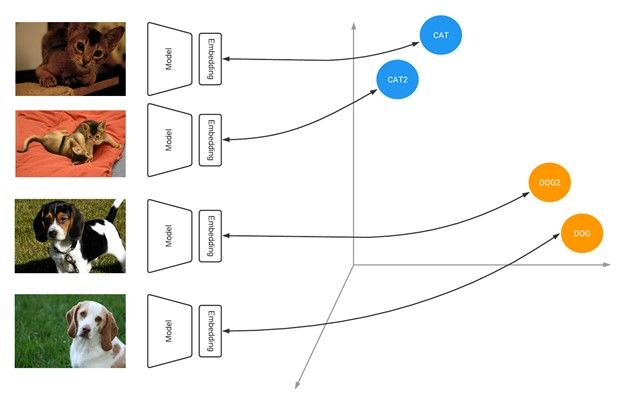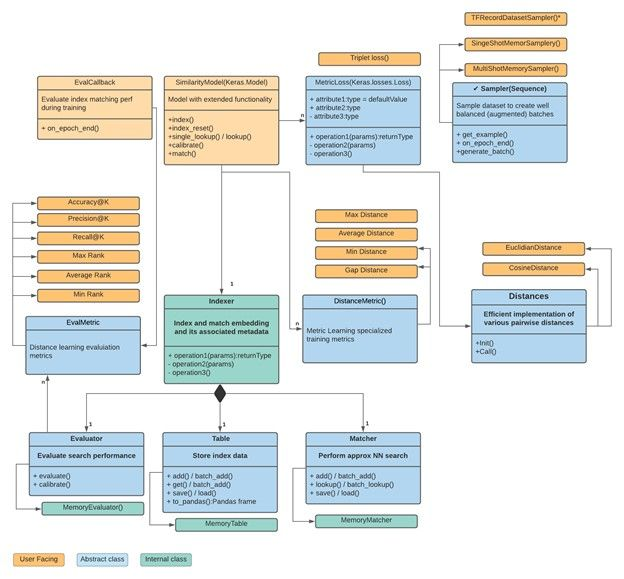Facilitating the Spread of Knowledge and Innovation in Professional Software Development

Write for InfoQ

### Topics

InfoQ Homepage News TensorFlow Similarity Supports Fast Query Search Index on Pre-trained Models

# TensorFlow Similarity Supports Fast Query Search Index on Pre-trained Models

This item in japanese

Keras framework creator Francois Chollet and his team recently released a Python library for TensorFlow, called TensorFlow Similarity, designed to make it easy to build similarity models.

Similarity learning is the process of finding similar items, from similar clothes in images to person identification using face pictures. Deep-learning models have used a method called contrastive learning to increase accuracy and efficiency in learning similarity between images. In contrastive-learning models, multiple and similar convolutional-networks architectures generate embedded feature vectors (vector output of a series of convolutions), which are fed into a contrastive loss evaluating/comparing  positive and negative cases of similarity between images. A simple example is identifying the same person's face in different pictures.

One of the advantages of TensorFlow Similarity is the fast query search index using pre-trained models. This means if you want to search dogs, you can provide an image to the API and it will search for similar items in the model database and retrieve the items in linear time with high accuracy.

Another advantage is the easy integration of new search categories to a model without the need to retrain it from scratch.

A code example for the MNIST dataset can be written in 20 lines:

from tensorflow.keras import layers
# Embedding output layer with L2 norm
from tensorflow_similarity.layers import MetricEmbedding
# Specialized metric loss
from tensorflow_similarity.losses import MultiSimilarityLoss
# Sub classed keras Model with support for indexing
from tensorflow_similarity.models import SimilarityModel
# Data sampler that pulls datasets directly from tf dataset catalog
from tensorflow_similarity.samplers import TFDatasetMultiShotMemorySampler
# Nearest neighbor visualizer
from tensorflow_similarity.visualization import viz_neigbors_imgs
# Data sampler that generates balanced batches from MNIST dataset
sampler = TFDatasetMultiShotMemorySampler(dataset_name='mnist', classes_per_batch=10)
# Build a Similarity model using standard Keras layers
inputs = layers.Input(shape=(28, 28, 1))
x = layers.Rescaling(1/255)(inputs)
x = layers.Conv2D(64, 3, activation='relu')(x)
x = layers.Flatten()(x)
x = layers.Dense(64, activation='relu')(x)
outputs = MetricEmbedding(64)(x)
# Build a specialized Similarity model
model = SimilarityModel(inputs, outputs)
# Train Similarity model using contrastive loss
model.fit(sampler, epochs=5)
# Index 100 embedded MNIST examples to make them searchable
sx, sy = sampler.get_slice(0,100)
model.index(x=sx, y=sy, data=sx)
# Find the top 5 most similar indexed MNIST examples for a given example
qx, qy = sampler.get_slice(3713, 1)
nns = model.single_lookup(qx)
# Visualize the query example and its top 5 neighbors
viz_neigbors_imgs(qx, qy, nns)Figure: Similarity models learn to output embeddings that project items in a metric space where similar items are close together and far from dissimilar ones Source.

Currently there are only supervised models available and the API is still in beta. Although any supervised model implemented in Keras.model can be used with this API, only EfficientNet is given as an example. EfficientNet is a highly efficient convolution-network architecture with an inference time 6.1x faster and 8.4x smaller than the best existing Conv-Net.

Nevertheless, only EfficientNet is implemented; you can create your own customized similarity model as follows:

def get_model():
inputs = layers.Input(shape=(28, 28, 1))
x = layers.experimental.preprocessing.Rescaling(1/255)(inputs)
x = layers.Conv2D(32, 7, activation='relu')(x)
x = layers.Conv2D(32, 3, activation='relu')(x)
x = layers.MaxPool2D()(x)
x = layers.Conv2D(64, 7, activation='relu')(x)
x = layers.Conv2D(64, 3, activation='relu')(x)
x = layers.Flatten()(x)
x = layers.Dense(64, activation='relu')(x)
# smaller embeddings will have faster lookup times while a larger embedding will improve the accuracy up to a point.
outputs = MetricEmbedding(64)(x)
return SimilarityModel(inputs, outputs)
model = get_model()
model.summary()


There are other non-official libraries for similarity learning like Pytorch Metric Learning which seems to require more knowledge for usage.

The community warmly welcomed this new TensorFlow tool with thousands of shares on Twitter.

The API is divided conceptually into similarity model, distance Metrics, distances and loss functions. The available loss functions available are Triplet Loss , PN Loss , Multi Sim Loss and Circle LossFigure: Tensorflow Similarity API chart flow Source.

Finally, the API code can be used with proper authors reference according to the TensorFlow Similarity Apache 2.0  repository LICENSE.

Style

## Hello stranger!

You need to Register an InfoQ account or or login to post comments. But there's so much more behind being registered.

Get the most out of the InfoQ experience.

Allowed html: a,b,br,blockquote,i,li,pre,u,ul,p